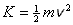This is the defining equation for the kinetic energy of a point particle. It states that the kinetic energy of a point particle is one-half times the mass of that particle times the square of the magnitude of its velocity.

Pitfall Avoidance Note: Make sure you don't mistake kinetic energy for the force constant of a spring and vice versa. Kinetic energy is represented by an upper case K. The force constant for a spring is represented by a lower case k.